# DC circuit calculations

## Electricity and Electronics

• #### Question 1

Calculate the amount of voltage between points {\bf A} and {\bf B} in this circuit. You must sketch polarity marks (+ , $-$) on the schematic diagram to show the polarity of $V_{AB}$, as well as show all of your mathematical work!• #### Question 2

Calculate the resistance between points {\bf A} and {\bf B} ($R_{AB}$) for the following resistor networks:• #### Question 3

Use Kirchhoff’s Voltage Law to calculate the magnitude and polarity of the voltage across resistors R_2 and R_4 in this resistor network:• #### Question 4

Imagine you are using a digital voltmeter to measure voltages between pairs of points in a circuit, following the sequence of steps shown in the following diagrams: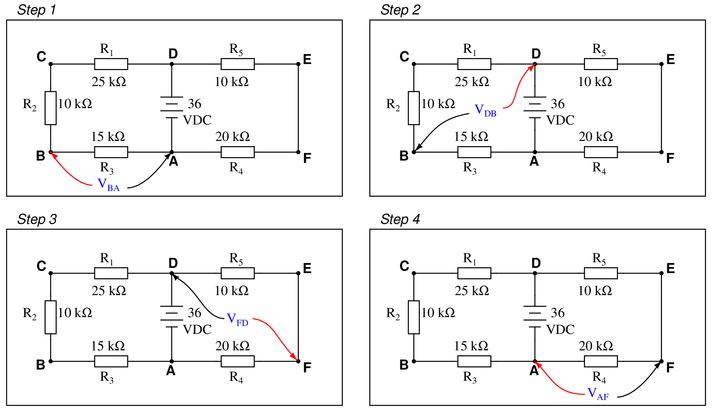How much voltage would be registered by the voltmeter in each of the steps? Be sure to include the sign of the DC voltage measured (note the coloring of the voltmeter leads, with the red lead always on the first point denoted in the subscript: $V_{BA}$ = red lead on B’’ and black lead on A’‘):

{\bullet} $V_{BA} =$
{\bullet} $V_{DB} =$
{\bullet} $V_{FD} =$
{\bullet} $V_{AF} =$

What is the algebraic sum of these voltages?

• #### Question 5

Calculate the amount of voltage dropped across resistor R_2:Also, note the direction of current through it and the polarity of the voltage drop across it.

• #### Question 6

Complete the table of values for this circuit:• #### Question 7

Suppose you were designing a circuit that required two LEDs for power on’’ indication. The power supply voltage is 15 volts, and each LED is rated at 1.6 volts and 20 mA. Calculate the dropping resistor sizes and power ratings:After doing this, a co-worker looks at your circuit and suggests a modification. Why not use a single dropping resistor for both LEDs, economizing the number of components necessary?Re-calculate the dropping resistor ratings (resistance {\it and} power) for the new design.

• #### Question 8

Complete the table of values for this circuit:• #### Question 9

Complete the table of values for this circuit:• #### Question 10

Complete the table of values for this circuit:• #### Question 11

Identify which of these components are connected directly in series with each other, and which are connected directly in parallel with each other:Assume that the open wire ends are connection points to a power source. In circuits where ground symbols appear, consider ground as the other side of the power source.

• #### Question 12

Complete the table of values for this circuit: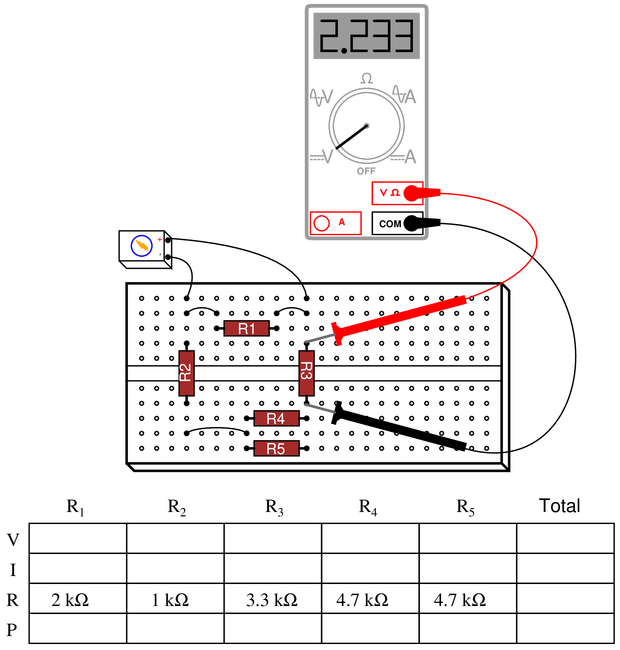• #### Question 13

When the 5 k$\Omega$ potentiometer in this circuit is set to its 0{\bullet} At 0
{\bullet} At 25
{\bullet} At 50
{\bullet} At 75
{\bullet} At 100

Calculate what the output voltages will be if a 1 k$\Omega$ load resistor is connected between the $V_{out}$’’ terminal and ground:{\bullet} At 0
{\bullet} At 25
{\bullet} At 50
{\bullet} At 75
{\bullet} At 100

• #### Question 14

Determine the voltages (with respect to ground) at points {\bf A} and {\bf B} in this circuit under four different conditions: both loads off, load 1 on (only), load 2 on (only), and both loads on:$$\begin{array} {|l|l|} \hline Voltage & Both loads off & Load 1 on (only) & Load 2 on (only) & Both loads on \\ \hline V_A & & & & \\ \hline V_B & & & & \\ \hline \end{array}$$

• #### Question 15

One of the resistors in this voltage divider circuit is failed open. Based on the voltage readings shown at each load, determine which one it is:• #### Question 16

One of the resistors in this voltage divider circuit is failed open. Based on the voltage readings shown at each load, determine which one it is:• #### Question 17

One of the resistors in this voltage divider circuit is failed (either open or shorted). Based on the voltage readings shown at each load, determine which one and what type of failure it is:• #### Question 18

Old vacuum-tube based electronic circuits often required several different voltage levels for proper operation. An easy way to obtain these different power supply voltages was to take a single, high-voltage power supply circuit and divide’’ the total voltage into smaller divisions.

These voltage divider circuits also made provision for a small amount of wasted’’ current through the divider called a {\it bleeder} current, designed to discharge the high voltage output of the power supply quickly when it was turned off.

Design a high-voltage divider to provide the following loads with their necessary voltages, plus a bleeder’’ current of 5 mA (the amount of current going through resistor R4):• #### Question 19

Calculate the necessary value of $R$ to create a voltage drop of 4 volts between test points {\bf A} and {\bf B}: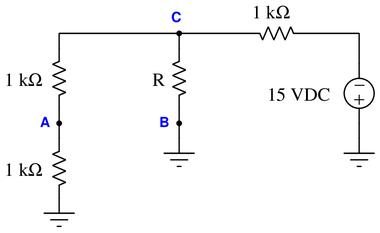• #### Question 20

In a parallel circuit, certain general principles may be stated with regard to quantities of voltage, current, resistance, and power. Complete these sentences, each one describing a fundamental principle of parallel circuits:

In a parallel circuit, voltage . . .’‘

In a parallel circuit, current . . .’‘

In a parallel circuit, resistance . . .’‘

In a parallel circuit, power . . .’‘

For each of these rules, explain {\it why} it is true.

• #### Question 21

Explain, step by step, how to calculate the amount of current ($I$) that will go through each resistor in this series circuit, and also the current ($I$) supplied by the DC voltage source:• #### Question 22

Determine the amount of voltage dropped by each resistor in this circuit, if each resistor has a color code of Brn, Blk, Red, Gld (assume perfectly precise resistance values—0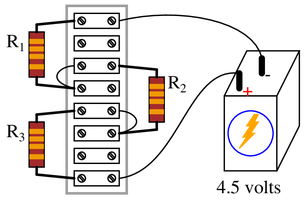{\bullet} Current through each resistor
{\bullet} Power dissipated by each resistor
{\bullet} Ratio of each resistor’s voltage drop to battery voltage ($E_R \over E_{bat}$)
{\bullet} Ratio of each resistor’s resistance to the total circuit resistance ($R \over R_{total}$)

• #### Question 23

Calculate the output voltages of these two voltage divider circuits ($V_A$ and $V_B$):Now, calculate the voltage between points {\bf A} (red lead) and {\bf B} (black lead) ($V_{AB}$).

• #### Question 24

Calculate both the maximum and the minimum amount of voltage obtainable from this potentiometer circuit (as measured between the wiper and ground):• #### Question 25

Suppose that an electric heater, which is nothing more than a large resistor, dissipates 500 watts of power when directly connected to a 110 volt source:Now suppose that exact same heater is connected to one end of a long two-wire cable, which is then connected to the same 110 volt source. Assuming that each conductor within the cable has an end-to-end resistance of 3 ohms, how much power will the heater dissipate?• #### Question 26

Suppose an analog voltmeter has a range of 0 to 10 volts, and an internal resistance of exactly 100 k$\Omega$: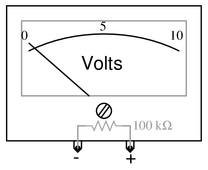Show how a single resistor could be connected to this voltmeter to extend its range to {\it 0 to 50} volts. Calculate the resistance of this range’’ resistor, as well as its necessary power dissipation rating.

• #### Question 27

Determine the voltages registered by a voltmeter between the following points in this circuit. Be sure to note whether the voltmeter’s indication will be a positive value or a negative value in each case:{V_A =} {\underline{ 40pt}} (red lead on {\bf A}, black lead on ground)

{V_B =} {\underline{ 40pt}} (red lead on {\bf B}, black lead on ground)

{V_C =} {\underline{ 40pt}} (red lead on {\bf C}, black lead on ground)

{V_D =} {\underline{ 40pt}} (red lead on {\bf D}, black lead on ground)

{V_{AC} =} {\underline{ 40pt}} (red lead on {\bf A}, black lead on {\bf C})

{V_{DB} =} {\underline{ 40pt}} (red lead on {\bf D}, black lead on {\bf B})

{V_{BA} =} {\underline{ 40pt}} (red lead on {\bf B}, black lead on {\bf A})

{V_{BC} =}{\underline{ 40pt}} (red lead on {\bf B}, black lead on {\bf C})

{V_{CD} =} {\underline{ 40pt}} (red lead on {\bf C}, black lead on {\bf D})

• #### Question 28

Calculate the amount of voltage between test points TP1 and TP3, and also the amount of voltage between test points TP2 and TP4:$V_{TP1-TP3}$ = 40pt $V_{TP2-TP4}$ =

• #### Question 29

In a series circuit, certain general principles may be stated with regard to quantities of voltage, current, resistance, and power. Complete these sentences, each one describing a fundamental principle of series circuits:

In a series circuit, voltage . . .’‘

In a series circuit, current . . .’‘

In a series circuit, resistance . . .’‘

In a series circuit, power . . .’‘

For each of these rules, explain {\it why} it is true.

• #### Question 30

Use Kirchhoff’s Current Law to calculate the magnitude and direction of the current through resistor R_4 in this resistor network: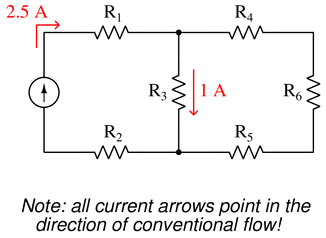• #### Question 31

Explain, step by step, how to calculate the amount of current ($I$) that will go through each resistor in this parallel circuit, and also the current ($I$) supplied by the DC voltage source:• #### Question 32

Calculate the total amount of current that the battery must supply to this parallel circuit:Now, using Ohm’s Law, calculate total resistance ($R_{total}$) from total (source) voltage $V_{total}$ and total (source) current $I_{total}$.

• #### Question 33

Complete the table of values for this circuit:• #### Question 34

Complete the table of values for this circuit:• #### Question 35

The circuit shown here is commonly referred to as a {\it current divider}. Calculate the voltage dropped across each resistor, the current drawn by each resistor, and the total amount of electrical resistance seen’’ by the 9-volt battery: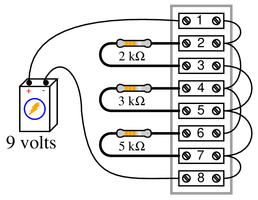{\bullet} Current through the 2 k$\Omega$ resistor =
{\bullet} Current through the 3 k$\Omega$ resistor =
{\bullet} Current through the 5 k$\Omega$ resistor =
{\bullet} Voltage across each resistor =
{\bullet} $R_{total}$ =

• #### Question 36

Examine these two variable-resistance ({\it rheostat}) networks, each one with a large-range potentiometer and a small-range potentiometer:For each network, determine which pot is the {\it coarse} adjustment and which pot is the {\it fine} adjustment for total network resistance, and explain your reasoning.

• #### Question 37

Identify which of these components are connected directly in series with each other, and which are connected directly in parallel with each other:Assume that the open wire ends are connection points to a power source.